A 3D quantitative evaluation for assessing the changes of treatment planning system and irradiation

A 3D quantitative evaluation for assessing the changes of treatment planning system and irradiation techniques in radiotherapy

1Department of Radiation Oncology, University Joseph Fourier, Grenoble, France.
2Department of Medical physics, Grenoble University Hospital, France.

Cite this article as: Chaikh A, Giraud JY, Balosso J. A 3D quantitative evaluation for assessing the changes of treatment planning system and irradia-tion techniques in radiotherapy. Int J Cancer Ther Oncol 2014; 2(3):02033.
DOI:
http://dx.doi.org/10.14319/ijcto.0203.3

Original Article

Abstract

Purpose: This work proposes and compares two 3D global evaluation methods for assessing the alteration of calculated dose distributions when treatment planning system algorithms or irradiation techniques is modified in radiation therapy.

Methods: The global analysis is based on gamma index (γ) proposed by Low et al.1 and Chi (χ) index proposed by Bakai et al.2. The γ and χ values are signed in order to identify the over and under estimating dosage. The 3D maps, the cumulative Gamma Voxels Histograms (GVHs) and Chi Voxels Histograms (CVHs) were generated using two software. The γ and χ criteria were set to 3 mm for the distance to agreement and 3% for dose. Pearson's Chi-squared test was applied to assess the statistically significance between GVHs and CVHs. We illustrated this method for the change of dose calculation algorithms for lung cancer, and the change of irradiation techniques for breast cancer. For each patient, 2 treatment plans were generated. For the example of change of dose calculation algorithms, a plan 1 was calculated using Pencil Beam Convolution (PBC) algorithm and a plan 2 was calculated using Modified Batho method (PBC-MB). For the example of change of irradiation technique, a plan 1 was calculated using Source Skin Distance SSD technique and a plan 2 was calculated using a single isocenter technique.

Results: The 3D analysis based on γ and χ indexes showed a significant effect on the dosimetric representation in the lung cancer when we change the PBC algorithm to PBC-MB method. The comparison between the two irradiation techniques showed that the single isocenter technique produces a better dose distribution for the treatment of breast cancer. Pearson's Chi-squared test showed that there was no statistically significance between GVHs and CVHs generated by γ and χ indexes, (p > 0.05). The global analysis using 3D for γ and χ indexes confirmed the results obtained from dosimetric analysis.

Conclusion: The methods proposed in this study provide useful tools for radiotherapy to compare two dose distributions obtained using different algorithms or different irradiation techniques. The χ-index was (~190) times faster than γ-index. The χ-index is thus a valuable and more convenient method for 3D global analysis compared with γ-index.

Keywords: Gamma Index; Dose Distributions; Gamma Voxels Histograms; Gamma Maps

Introduction

The optimization of irradiation therapy is a continuous change from reference Treatment Planning System (TPS) or irradiation techniques to novel algorithms and irradiation techniques. The switch from the reference treatment plan to the new one deserves caution to ensure that the new treatment will give the expected same clinical outcome. Therefore, the implementation of a new technique implies a dosimetric assessment. Traditionally, the treatment plans were evaluated by comparing the dose volume histograms and dose distributions. This process is complex and time consuming. In order to make this process easier and quantitative, we propose to consider two 3D global analysis using gamma (γ) index proposed by Low et al.1 and Chi (χ) index proposed by Bakai et al.2 These methods were carried out for two types of technical changes. First we assessed the impact of switching from dose calculation algorithm without density correction to an algorithm which takes into account the density correction for lung cancer treatment. Second, we assessed the impact of switching from the Source Skin Distance SSD technique to the single isocenter technique for breast cancer treatment.

Methods and Materials

Global analysis method

The original γ-index was introduced by Low et al.1, to compare and evaluate the dose distribution in 2D and 3D.1 The γ-index has been revised and improved for better accuracy and efficiency Bakai et al.2 Later, Gamma Volume Histograms (GVHs) for 2D and 3D were introduced by Spezi et al. to compare IMRT treatment plans.3 In our study we used the γ-index proposed by Low et al.1, and χ-index proposed by Bakai et al. 2. Both indexes are particularly useful when making an overall comparison of all fields used in each treatment plan. These indexes combine two criteria to compare tested and reference points: the Distance to Agreement (DTA) in millimeters and the dose difference in percentage.

For γ-index an ellipse is used to determine the acceptance region. The equation (1) shows the γ-index proposed by Low et al.1: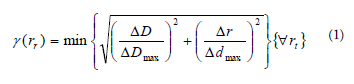ΔDmax and Δdmax were maximum acceptable dose difference and maximum acceptable DTA. ΔD = Dt - Dr is the dose difference between tested dose (Dt) and reference dose (Dr). Δr = rt - rr is the difference of distance between tested and reference points. The γ≤ 1 represents fulfillment of the criteria.

Bakai et al. 2, used the tube concept to make the evaluation process more efficient. This means the acceptance ellipses used in the gamma index became a tube that followed the reference distribution. The acceptance criteria at a reference point were determined using the local dose gradient, the dose difference and the DTA criteria. The value of χ-index was the ratio of the dose difference to the locally determined acceptance criteria. The equation 2 shows the χ-index provided by Bakai et al. 2: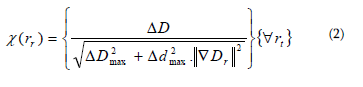ΔDmax and Δdmax were maximum dose and maximum distance criteria, respectively. ΔD and ▽Dr were the dose difference and the local dose gradient, respectively. The |χ| ≤ ± 1 represents the fulfillment of the criteria.

Evaluation of dose distribution in 3D

The DICOM images for each patient were exported from Eclipse® TPS (Version 8.1; Varian Medical Systems, Palo Alto, CA) for reference and tested plans including the dose distributions. The center, size and resolution of the dose matrix in the XYZ directions were defined using Eclipse® TPS. The anatomical position of the treatment isocenter was used as the reference point. The 3D dose evaluation was displayed using γ and χ maps along with the cumulative GVHs and CVHs which indicates the fraction of voxels with a γ and χ indexes equal or lower than a specific value. Figure 1 shows the schematic of the 3D evaluation using γ and χ indexes.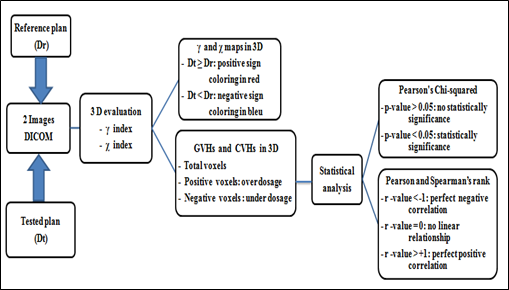FIG. 1: Schematic of the 3D evaluation using γ and χ indexes with statistical analysis.

For this work two software were developed: one software was used for compute the γ maps and GVHs, and this software has been adapted to compute the χ maps and CVHs. Both software’s analyze all voxels in the DICOM images and sort the total voxels detected in the DICOM images into positive and negative values. If the difference between the reference and tested plans induced an absolute value of γ and χ indexes above 1, this will demonstrate either an under dosage or over-dosage. However, in order to discriminate an over from an under estimated dose, a sign was attributed to absolute values of γ and χ indexes:

• If Dt ≥ Dr, the positive sign was attributed to the absolute value for γ and χ indexes. This shows over dosage indicating that the calculated dose with the tested plan is superior to the calculated dose with the reference plan.
• If Dt < Dr, the negative sign was attributed to the absolute value for γ and χ indexes. This shows under dosage indicating that the calculated dose with the tested plan is inferior to the calculated dose with the reference plan.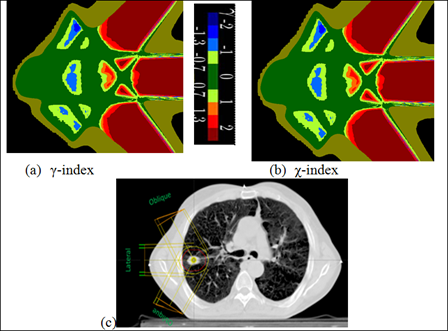FIG. 2: For lung cancer, a sample of γ and χ maps in 3D plotted in the transverse plan for comparing dose calculation algorithms for case 1(graphs a and b). The image in the left shows γ maps based on Low et al.1 and the image in the right shows χ maps based on Bakai et al.2. The red and blue coloring indicates that gamma is outside the tolerance criteria with over or under estimation of the dose respectively. Kaki coloring indicates the pixels with doses lower than 10% of the prescribed dose. Black coloring indicates the voxels ~ 0Gy. Graph (c) shows the CT scan for the parenchyma site; the red circle shows PTV2 and the yellow circle shows PTV1.

In this study all voxels in the DICOM image covering both the Planning Targets Volumes (PTVs) and the organs at risks (OARs) were included. Voxels outside the patient image (~ 0 Gy) were ignored (black pixels in the spatial distribution figures). Voxels with lower dose (10%) were also disregarded for the quantitative analysis as the relative dose threshold is easily met and generate a bias in the result (kaki pixels), as shown in Figures 2 and 5.

• γ and χ maps in 3D: the maps were displayed with three color coded regions: green indicates γ and χ values ranging from -1 to +1; red shows the γ and χ values ranging from +1 to +2; and blue shows γ and χ values ranging from -1 to -2. Thus the blue and red zones show respectively under or overestimated dose resulting from tested plan compared with reference plan.
• Cumulative GVHs and CVHs in 3D: the results of γ and χ maps for tested and reference plans were exploited for quantitative analysis by generating the cumulative GVHs and CVHs. The GVHs and CVHs show the volume ratios with a γ or χ indexs equal or lower than a given value. We considered that there was no dose difference between tested and reference plans, if 95% of voxels had |γ| and |χ| indexes ≤ 1.

In this study we used the 3D evaluation for γ and χ indexes to compare the spatial dose distribution for all structures: PTVs and OARs. The superposition of the γ and χ maps with the CT-Scan introduced the anatomic information. We could then see the healthy tissues located around the PTVs. The classical tolerance threshold of the gamma index in IMRT treatment plans quality control were used, i.e. 3% of dose and 3 mm for DTA.

Statistical analysis

The ratio of voxels having (|γ| ≤ 1) for the total, positive and negative GVHs were compared to that observed using χ-index. Pearson's Chi-squared test for 2 degrees of freedom was applied to assess the statistically significance between GVHs and CVHs generated by γ and χ indexes. Language R (version 2.15.2/2012-10-26) was employed to calculate p-value.4 The alpha error showing the probability to reject a true null hypothesis was fixed at 5%. A p-value < 0.05 was considered as statistically significant. To measure the relationship between the GVHs and CVHs resulting from γ and χ indexes, Pearson and Spearman’s rank correlation test was used. The results of test are between -1 and + 1:

• A result of -1 means that there is a perfect negative correlation between the values of GVHs and CVHs for all cases.
• A result of 0 means that there is no linear relationship between the values of GVHs and CVHs.
• A result of +1 means that there is a perfect positive correlation between the values of GVHs and CVHs for all cases.

It is very rare to get a correlation 0, -1 or +1, it usually comes out with something in between.5

Application of global analysis method
Comparison of dose calculation algorithms

We assess the impact of switching from Pencil Beam Convolution algorithm (PBC) integrated in the Eclipse® TPS (Version 8.1; Varian Medical Systems, Palo Alto, CA) to Modified Batho method (PBC-MB). PBC is a convolution algorithm based on pencil beam and calculates the dose without taking into account the heterogeneity correction.6, 7 PBC-MB method calculates the dose taking into account the 1D heterogeneity using a correction factor. The correction factor was determined by ray tracing from the primary radiation source to the point of interest.8, 9 PBC and PBC-MB were two different options in the use of Eclipse® TPS, i.e., switching the inhomogeneity correction on or off.

Treatment plans: For each patient, 2 treatment plans were generated using exactly the same configuration of beams, collimator and accessories:

• Plan 1: the dose was calculated using PBC algorithm.
• Plan 2: the dose was calculated using PBC-MB method.

Plan 1 was the reference plan and plan 2 was the tested plan. The reference plan was the one used to actually treat the patients and was designed according to the clinical experience of the department and ICRU recommendations. For the PTVs, 95% of the prescribed dose encompassed the volume and the maximum dose within the PTVs was under 107% of the prescribed dose. For organs at risk, the recommended dose constraints were respected in reference plan.

Clinical cases: 3 Patient records were used to evaluate the impact of switching from PBC algorithm to PBC-MB. The patients were treated using the 3D radiotherapy irradiation technique with 18MV photon beams. Radiation oncologists delineated the anatomic borders of target structures and OARs. The PTVs were defined according to the recommendations of ICRU Reports 50 and 62.10,11

• Case 1: 6 fields were used to treat a tumor in right lung parenchyma. The PTV received 66Gy.
• Case 2: 17 fields were used to treat a tumor located in mediastinum in left lung. The PTVs received 66Gy.
• Case 3: 8 fields were used to treat a tumor in esophagus. The PTV received 54Gy.

We assess the impact of switching from SSD technique to the single isocenter technique in the case of breast cancer treatment.

Treatment plans: for each patient, 2 treatment plans were generated and the dose was calculated using PBC-MB method for both irradiation techniques:

• Plan 1: the SSD technique was used.
• Plan 2: the single isocenter technique was used.

Plan 1 was thus the reference plan and plan 2 the tested plan. Plan 1 was used to actually treat the patients. The reference treatment plans were designed according to the clinical experience of the department and ICRU recommendations.

Clinical cases: 3 Cases were chosen representing typical irradiation situations: breast alone, breast and boost areas, breast and supra-clavicular areas. The patients were treated using the 3D radiotherapy irradiation technique with 6MV photon beams. Radiation oncologists delineated the anatomic borders of target structures and OARs.

• Case 1: 4 tangential fields were used to treat a tumor in left breast. The PTV received 50.6Gy.
• Case 2: 4 tangential fields were used to treat a tumor in left breast and the boost area. The PTVs received 10Gy.
• Case 3: 5 tangential fields were used to treat a tumor in left breast and supra-clavicular areas. The PTVs received 50.6Gy.

Results

Comparison of dose calculation algorithms Analysis of γ and χ maps in 3D
The comparison of spatial distribution using γ and χ maps showed that the two indexes produce values varying from 0 to │7│. The results of the 3 patients showed that using PBC-MB method the dose for OARs was superior (red coloring), but a fraction of the PTVs will be under dosage (blue coloring) compared to reference PBC algorithm. Figure 2 (a) and 2 (b) show a sample of γ and χ maps resulting from the comparison between the two algorithms combining reference and tested plans for patient 1. It can be seen that both indexes showed the same maps. Figure 2 (c) shows the CT scan for the parenchyma site; the red circle shows PTV2 and the yellow circle shows PTV1. It is clear in Figure 2, that PBC and PBC-MB are different for large areas where γ and χ are > 1 outside of the PTV. We observed that inside the PTV, only small portions showed a difference between PBC and PBC-MB leading to a γ and χ > 1.

Analysis of cumulative GVHs and CVHs in 3D
Table 1 shows the total, positive and negative voxels calculated using γ and χ indexes for all clinical cases. In Table 1, it seems clear that, the ratio of positive voxels calculating with γ and χ indexes was inferior to the ratio of negative voxels. This indicates that using the PBC algorithm the PTVs will receive more dose than with PBC-MB method. Figure 3 shows the absolute values of the cumulative GVHs and CVHs using γ and χ indexes. They show the total, positive and negative voxels which discriminate the under and over dosage. It can be seen that 85% and 95% of the negative and positive voxels have a γ ≤ 1, respectively using γ and χ indexes. This shows under estimated doses when we switch from PBC to PBC-MB.

TABLE 1: Ratio of voxels with γ and χ ≤ 1 resulting from the comparison of PBC algorithm with PBC-MB method. The p-value was calculated using Pearson's Chi-squared test resulting from the comparison of γ and χ indexes.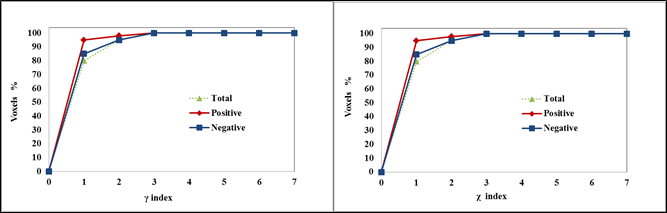FIG. 3
: Cumulative GVHs in 3D obtained when comparing the two algorithms for case 2. The image in the left shows γ-index based on Low et al. 1 and the image in the right shows χ-index based on Bakai et al. 2. It can be seen that 85% and 95% of the negative and positive voxels have a γ ≤ 1, respectively using γ and χ indexes. This means that the tolerance level of 95% of voxels having gamma ≤ 1 is not obtained for the negative voxels using PBC-MB method for plan 2.

Statistical analysis: Pearson's Chi-squared test showed that there was no statistically significant difference between γ and χ indexes, (p > 0.05), as shown in Table 1. Figure 4 shows the correlation between GVHs and CVHs for all voxels in all clinical cases. It can be seen that the correlations coefficient using Pearson and Spearman’s was r = 1 for positive and total voxels, but r = 0.99 for negative voxels. The correlation coefficient value shows a strong positive correlation between the two indexes. This means the values of voxels obtained using γ and χ were very similar.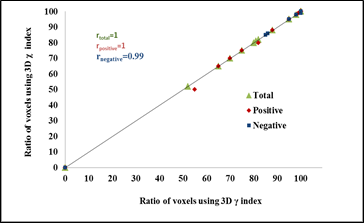FIG. 4: Correlation between γ GVHs index based on Low et al. 1, and χ GVHs based on Bakai et al. 2, for all voxels when comparing the two algorithms for all clinical cases. It can be seen that the correlation coefficient using Pearson and Spearman’s was r = 1 for positive and total voxels, but it was r = 0.99 for negative voxels.

Analysis of γ and χ maps in 3D

The comparison of spatial distribution using gamma γ and χ maps showed that the two indexes produce values varying from 0 to │7│. The results showed that using single isocenter technique the dose for PTVs was superior for cases 2 and 3. The Figure 5 shows a sample of γ and χ maps resulting from the comparison between the two techniques combining reference and tested plans for case 1. The result shows that the both index showed the same gamma maps. It is clear in Figure 5, that both techniques are different for large areas where γ and χ are > 1 inside the PTV. The red coloring shows more doses in the PTV using the single isocenter technique.

TABLE 2: Ratio of total, positive and negative voxels with γ and χ ≤ 1 resulting from the comparison of SSD technique with single isocenter technique. The p-value was calculated using Pearson's Chi-squared test resulting from the comparison of γ and χ indexes.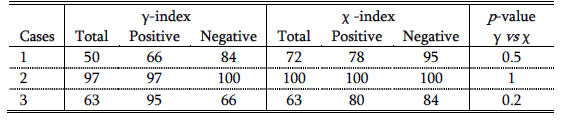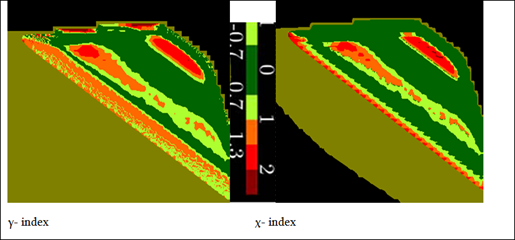FIG. 5: For breast treatment, a sample of γ and χ maps in 3D plotted in the transverse plan for comparing dose calculation algorithms for case 1. The image in the left shows γ maps based on Low et al.1 and the image in the right shows χ maps based on Bakai et al.2. The red coloring indicates that gamma is outside the tolerance criteria with over estimation of the dose. Kaki coloring indicates the pixels with doses lower than 10% of the prescribed dose. Black coloring indicates the voxels ~ 0Gy.

Analysis of Cumulative GVHs AND CVHs in 3D
Table 2 shows the total, positive and negative voxels calculated using γ and χ indexes for all clinical cases. The Table 2 clearly shows that the ratio of positive voxels calculated with γ and χ indexes was superior to the ratio of negative voxels for cases 2 and 3. This indicates that using the single isocenter technique the PTVs will receive more dose than with the SSD technique. The Figure 6 shows the absolute values of the cumulative GVHs and CVHs using γ and χ indexes for case 2. They show the positive, negative and total voxels which discriminate the under and over dosage. It can be seen that 97% and 100% of the positive and negative voxels have a γ ≤ 1, respectively. Conversely, 100% of the positive and negative voxels have a χ ≤ 1. In this case the tolerance level of 95% of voxels having γ or χ ≤ 1 was respected for negative and positive voxels for both indexes.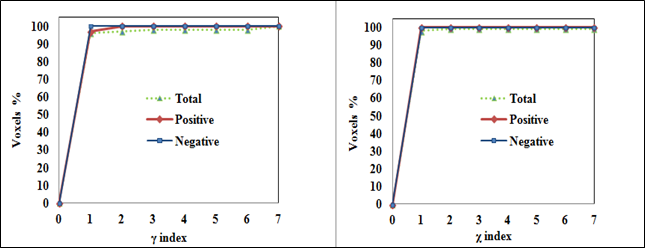FIG.6: Cumulative GVHs in 3D obtained when comparing SSD technique with single isocenter technique for case 2. The image in the left shows γ-index based on Low et al. 1 and the image in the right shows χ-index based on Bakai et al.2. It can be seen that 97% and 100% of the positive and negative voxels have a γ ≤ 1, respectively. Conversely, 100% of the positive and negative voxels have a χ ≤ 1.This means that the tolerance level of 95% of voxels having gamma ≤ 1 was respected for positive and negative voxels using SSD and single isocenter technique.

Statistical analysis: Pearson's Chi-squared test showed that there was no statistically significant difference between γ and χ indexes, (p > 0.05), as shown in Table 2. The Figure 7 shows the correlation between GVHs and CVHs for all voxels in all clinical cases. It can be seen that the correlations coefficient using Pearson and Spearman’s was r = 0.97 for positive and total voxels, but r = 0.99 for negative voxels. The correlation coefficient value shows a strong positive correlation between the two indexes. This means that the values of voxels obtained using γ and χ were very similar.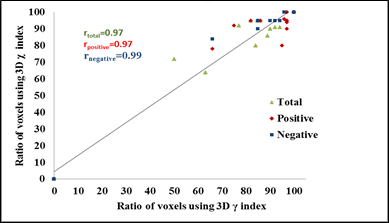FIG. 7: Correlation between γ GVHs index based on Low et al.1 and χ GVHs based on Bakai et al.2 for all voxels when comparing SSD technique with single isocenter technique for all clinical cases. It can be seen that the correlations coefficient using Pearson and Spearman’s was r = 0.97 for positive and total voxels, but was r = 0.99 for negative voxels.

Discussion

Currently, the DVH and isodose distribution, which result of decades of clinical experience, are used to compare and validate the treatment planning. We suggest in this study the use of (γ and χ) maps and cumulative (GVHs and CVHs) to objectively validate new treatments conditions, both dosimetric calculation and set up improvement. The γ and χ indexes provide an overall comparison that makes an easier comparison for 3D dose distribution. The advantage of 3D global analysis is that we can determine the under or overestimated dose resulting from the tested plan compared to reference plan. This method requires only DICOM images including the dose distribution. The superposition of γ or χ maps with the CT scan shows the anatomical structures. It is very convenient to verify the protection of OARs. The γ and χ maps provide a visual comparison of dose distribution for PTVs and OARs. The γ and χ values in the maps can be displayed in various color codes for easier interpretation. The comparison of positive and negative GVHs and CVHs allows qualifying if there are under or over dosage for any anatomical structures. Using the 3D evaluation the physicists could easily improve the treatment plan to protect the healthy tissues if there is any fraction of the voxels with (γ or χ >1 indicating over dosage), located in the region of interest. However, some of the clinical cases showed a less difference between γ and χ indexes (Table 2 and Figure 5). This difference is due to false positive or false negative for γ analysis.12

In this study we observed that the evaluation in 3D using γ and χ indexes is well adapted to compare two algorithms or two irradiation techniques. They showed a disagreement between the tested and reference plans. For the comparison of dose calculation algorithms, the γ and χ analysis demonstrated an under-dosage using PBC-MB method compared to reference PBC algorithm. Chaikh et al. showed that the risk due to the change in the algorithm from PBC to PBC-MB was a reduction in the dose delivered to the PTVs.13 In this study the 3D quantitative analysis based on γ and χ indexes confirms the same results obtained using the traditional dosimetric analysis by Chaikh et al.13. For the comparison of irradiation techniques, Chaikh et al. demonstrated that the usual dosimetric and statistical analysis (DVH, isodose curves, and quality indexes) were to the benefit of the single isocenter technique.14 The γ and χ analysis have confirmed this results.

We observed in this study that γ-index introduced by Low et al.1, was very time-consuming (~2 days) to compute γ maps in 3D. But, χ-index introduced by Bakai et al.2, was very less time-consuming (~15 min) to compute χ maps in 3D. This means that the χ-index evaluation was (~ 190) times faster than γ-index evaluation. Therefore, the use of γ or χ evaluation allow to following the irradiation of the patient during the treatment. The γ and χ indexes require only two DICOM images. To follow the patient irradiation, the reference image (Dr) based on the initial planning and the tested image (Dt) could be obtained during each treatment session. This needs the transfer of the fluence map of irradiation delivered by the linac, a very accurate re-positioning of the patient on the treatment table, and an identical treatment isocenter. As a whole, the χ indexes maps could be used for adaptive dosimetry approaches.

Conclusion

The γ and χ indexes provided a quantitative evaluation in 3D using the maps and cumulative GVHs and CVHs. The two indexes showed the same results (p > 0.05). The χ-index based on Bakai et al. 2, was computationally efficient compared with the original γ-index. The χ-index is a useful and rapid tool to compare the dose distribution in 3D. We propose the χ index for quantitative evaluation of dose distribution in 3D.

Conflict of Interest

The authors declare that they have no conflicts of interest. The authors alone are responsible for the content and writing of the paper.

Received March 17, 2014; Revised April 28, 2014; Accepted April 28, 2014; Published Online May 04, 2014

Corresponding author: Abdulhamid Chaikh; Department of Radiation Oncology, University Joseph Fourier, Grenoble, France.